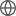Old Web
English
We present torus modelling for the X-ray spectra of a nearby narrow-line Seyfert 1 galaxy Mrk 1239 ($z=0.0199$), based on archival Suzaku, NuSTAR and Swift observations. Our model suggests very soft intrinsic power-law continuum emission of $\Gamma\approx2.57$ in 2019 and $\Gamma\approx2.98$ in 2007. By applying a correction factor to the unabsorbed X-ray luminosity, we find that Mrk 1239 is accreting near or around the Eddington limit. Our best-fit spectral model also suggests a torus with a column density of $\log(N_{\rm H, ave}/$cm$^{-2})=25.0\pm0.2$ and a high covering factor of $0.90$ in Mrk 1239, indicating that this source is most likely to be viewed almost face-on with $i\approx26^{\circ}$. Our line of sight might cross the edge of the torus with $N_{\rm H, los}=2-5\times10^{23}$cm$^{-2}$. The high Eddington ratio and the high line-of-sight column density makes Mrk 1239 one of the AGNs that are close to the limit where wind may form near the edge of the torus due to high radiation pressure.